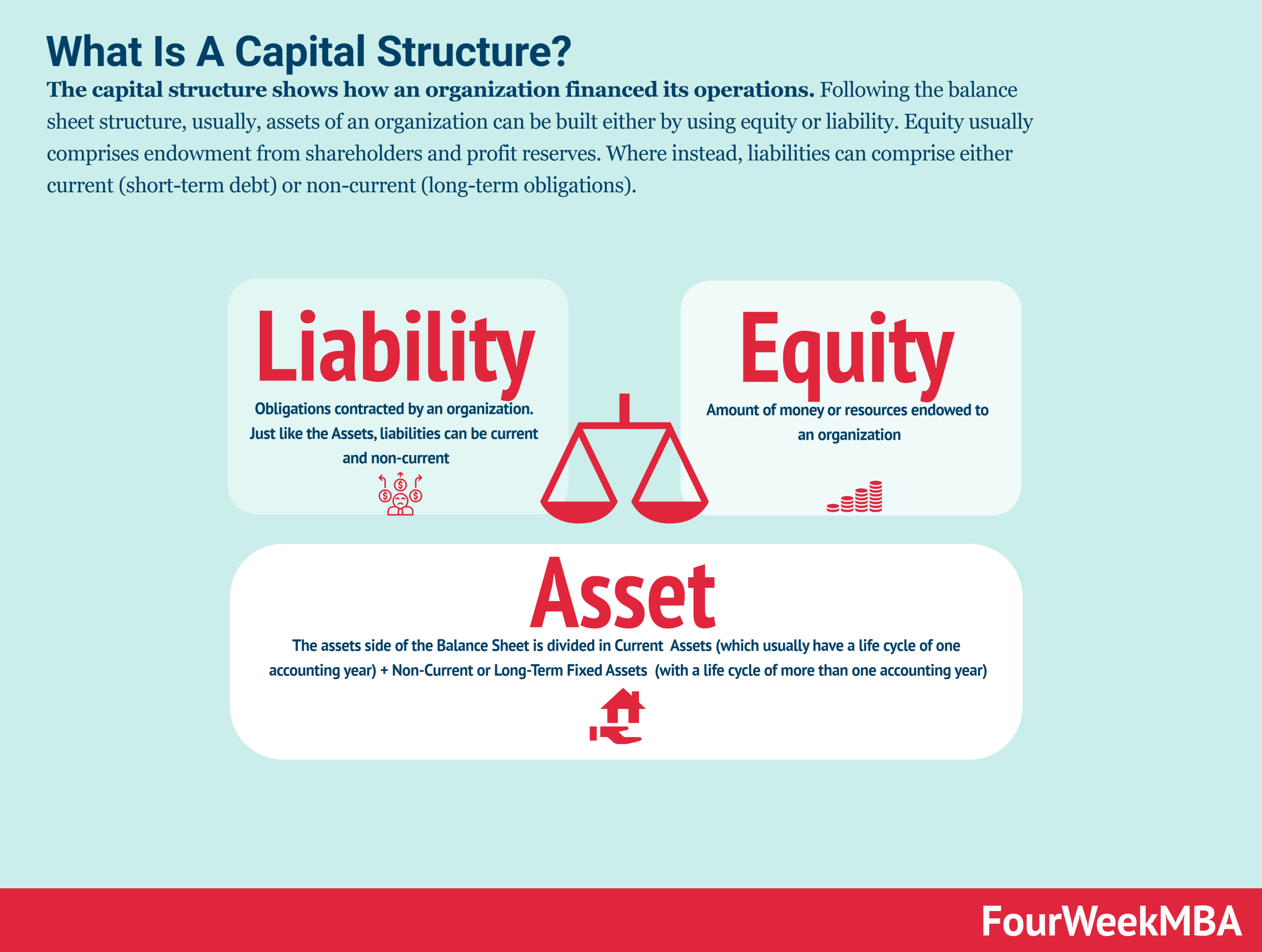The solvency ratios also called leverage ratios to help to assess the short and long-term capability of an organization to meet its obligations. Those ratios are critical to understanding whether the portion of debt held by the company can be sustained in the long run.

This ratio explains how much debt was used in acquiring the company’s assets and it is expressed either in number or percentage. The formula is:

Total liabilities / Total Assets

Imagine your coffee shop shows on the balance sheet \$200K of total liabilities and \$50K of equity. How do we compute the debt to asset ratio?

1. Compute the total assets: \$200K of liabilities + \$50K of equity = \$250K.

2. Compute the debt to asset ratio: \$200 of liabilities / \$250 of total assets = 0.8.

This means that 80% of the company’s assets have been financed through debt. A ratio lower than 0.5 or 50% indicates a fair level of risk. A ratio higher than 0.5 or 50% can determine a higher risk of the business. Of course, this ratio needs to be assessed against the ratio from comparable companies.

Debt to assets also explains how is the capital structure of the organization.The capital structure shows how an organization financed its operations. Following the balance sheet structure, usually, assets of an organization can be built either by using equity or liability. Equity usually comprises endowment from shareholders and profit reserves. Where instead, liabilities can comprise either current (short-term debt) or non-current (long-term obligations).
Scroll to Top
[class^="wpforms-"]
[class^="wpforms-"]